Binary Operations

Chapter 1 Class 12 Relation and Functions
Serial order wise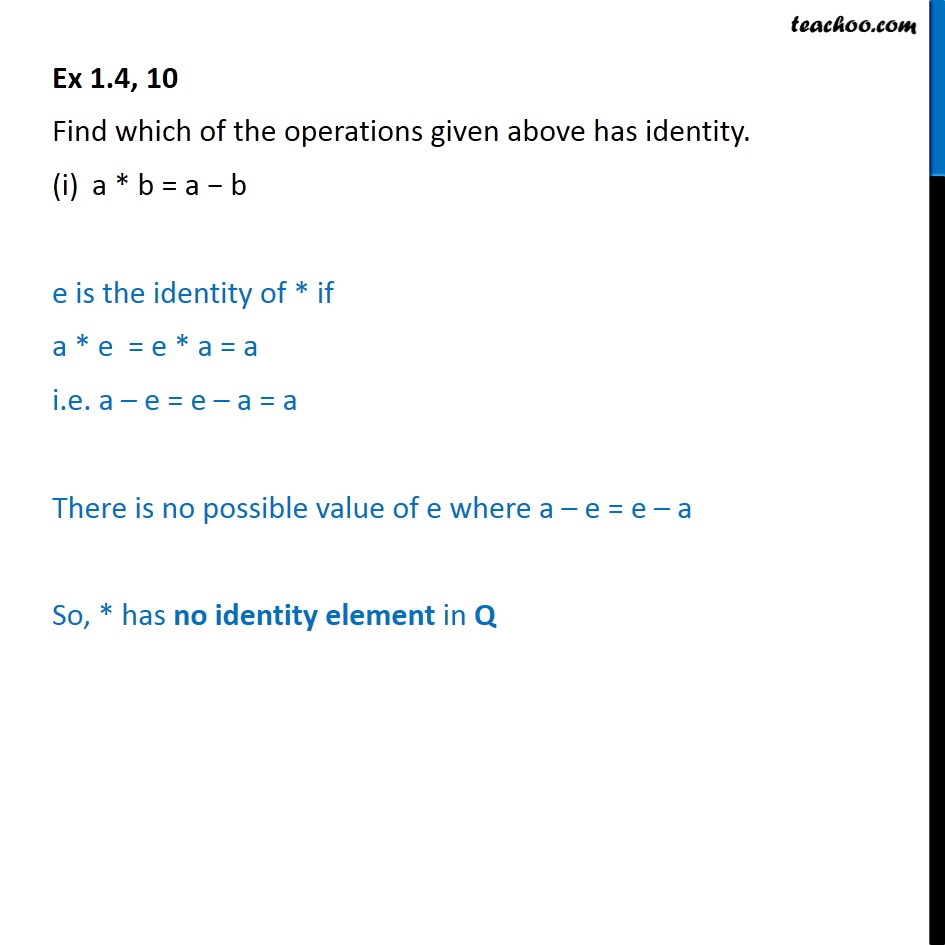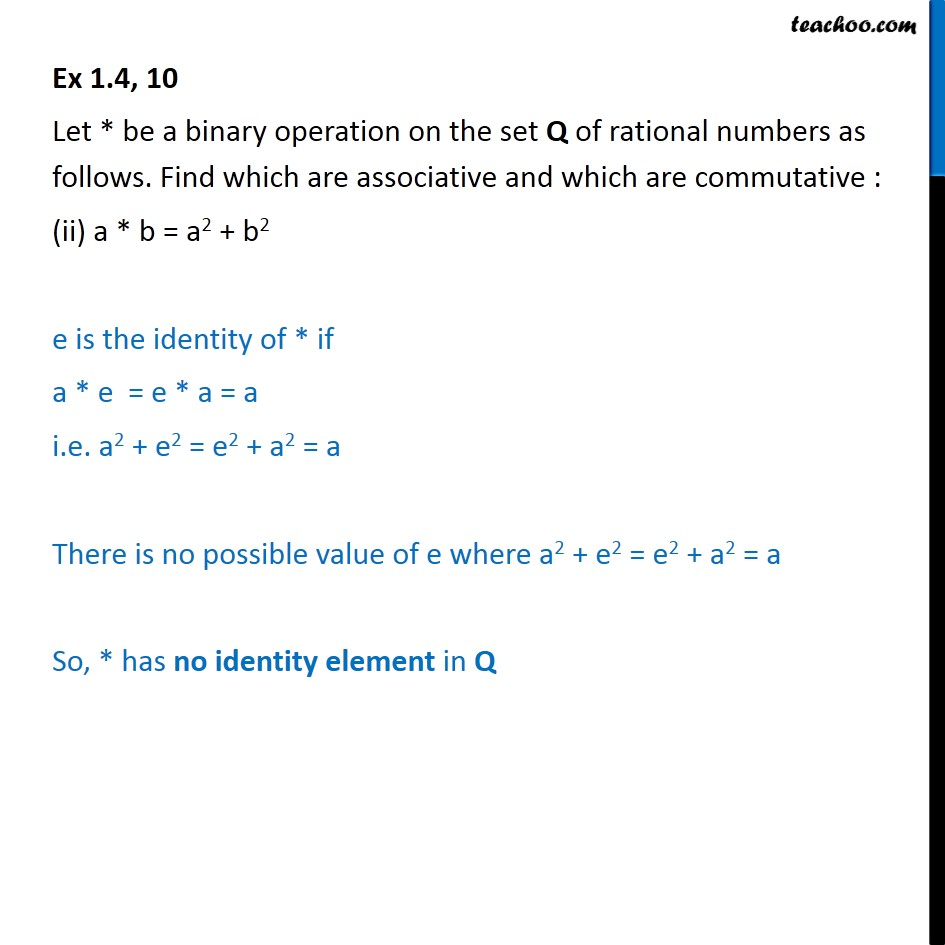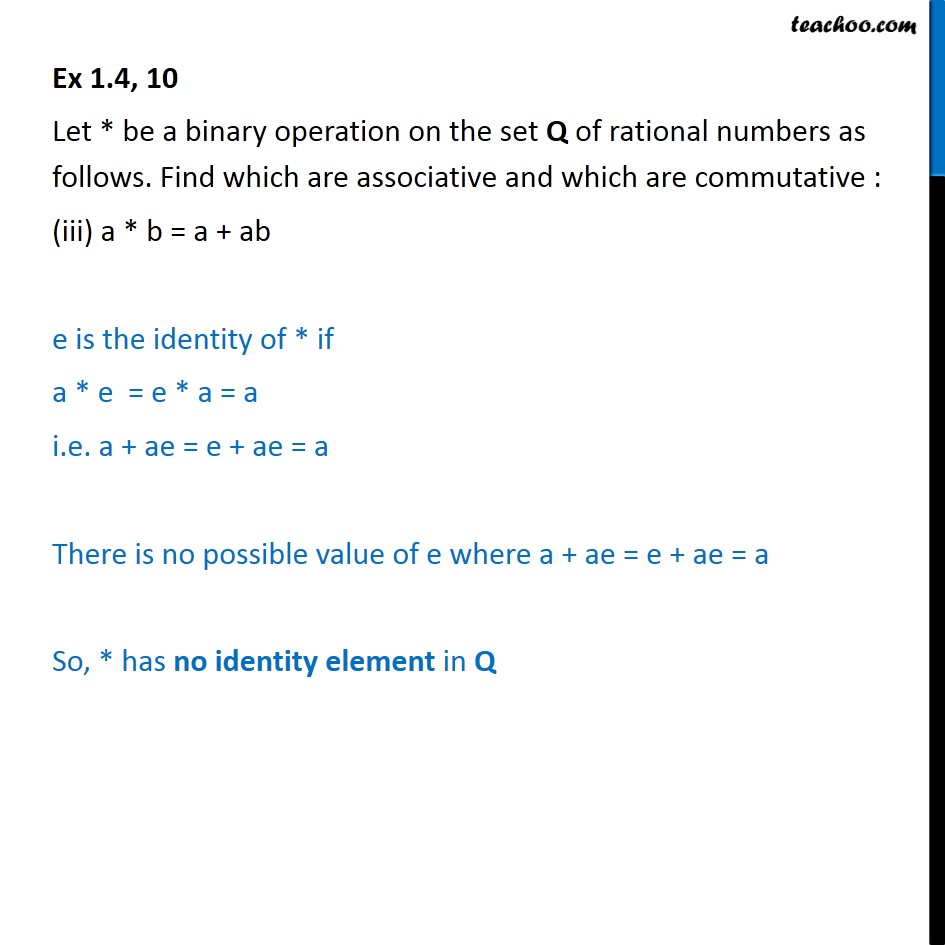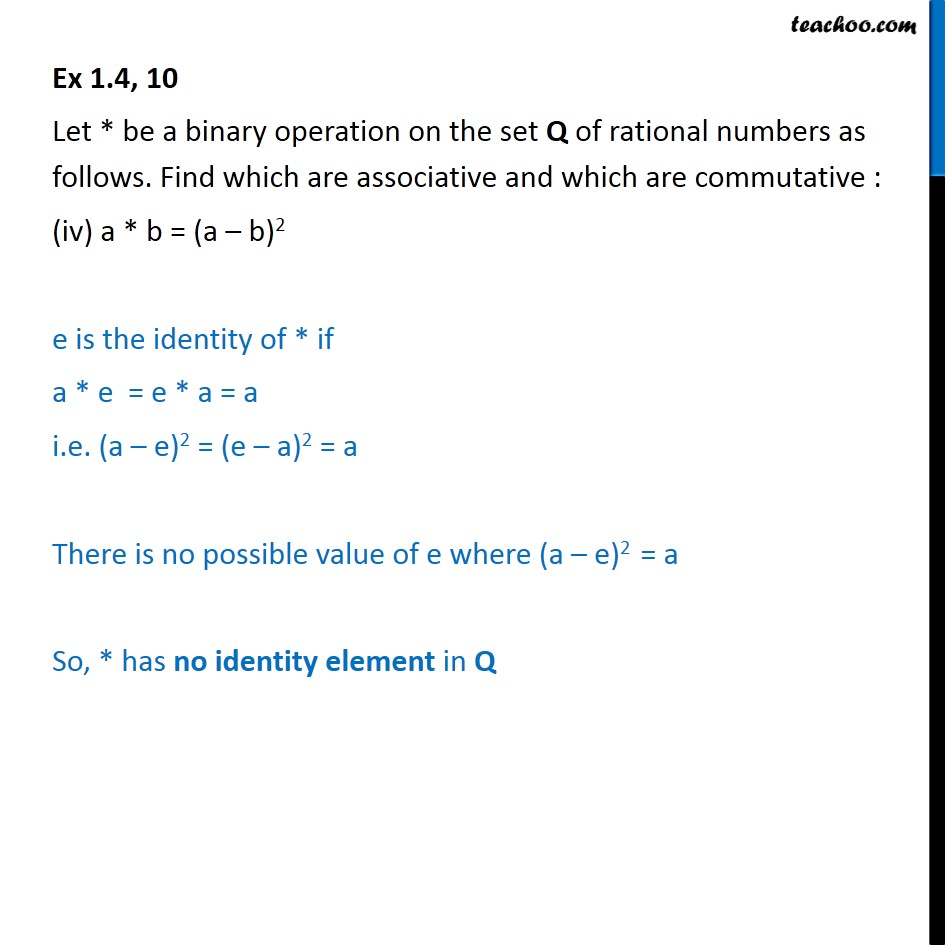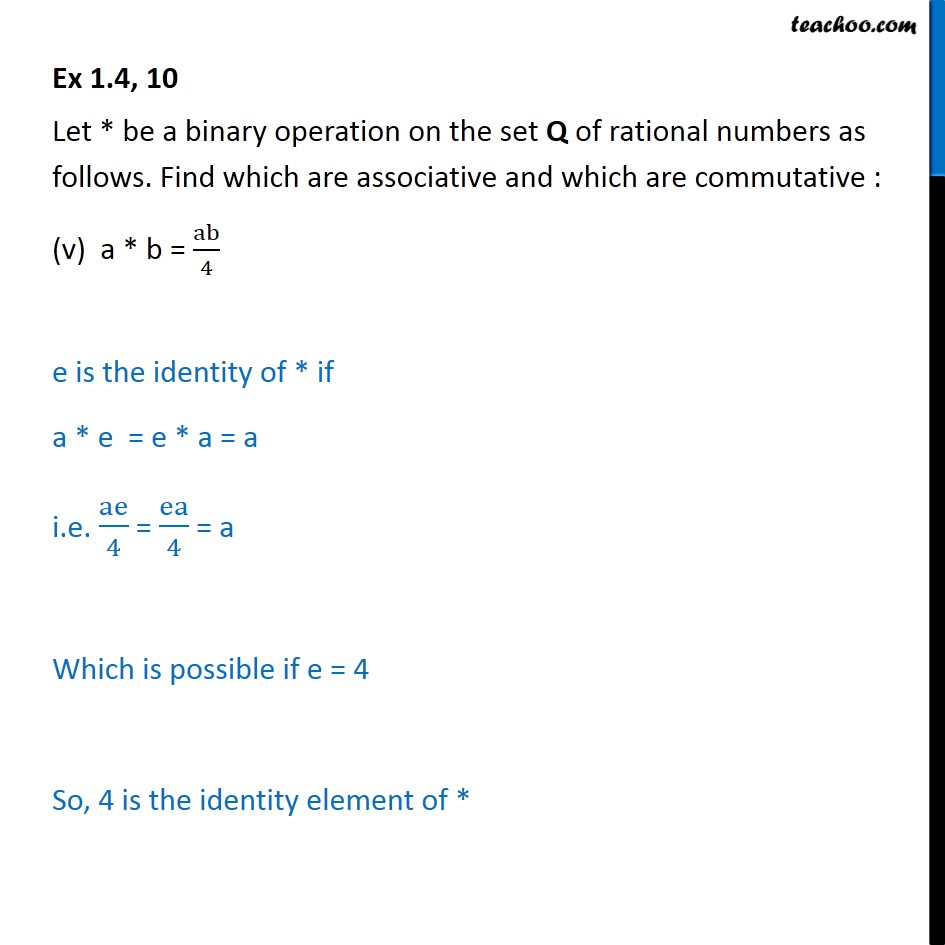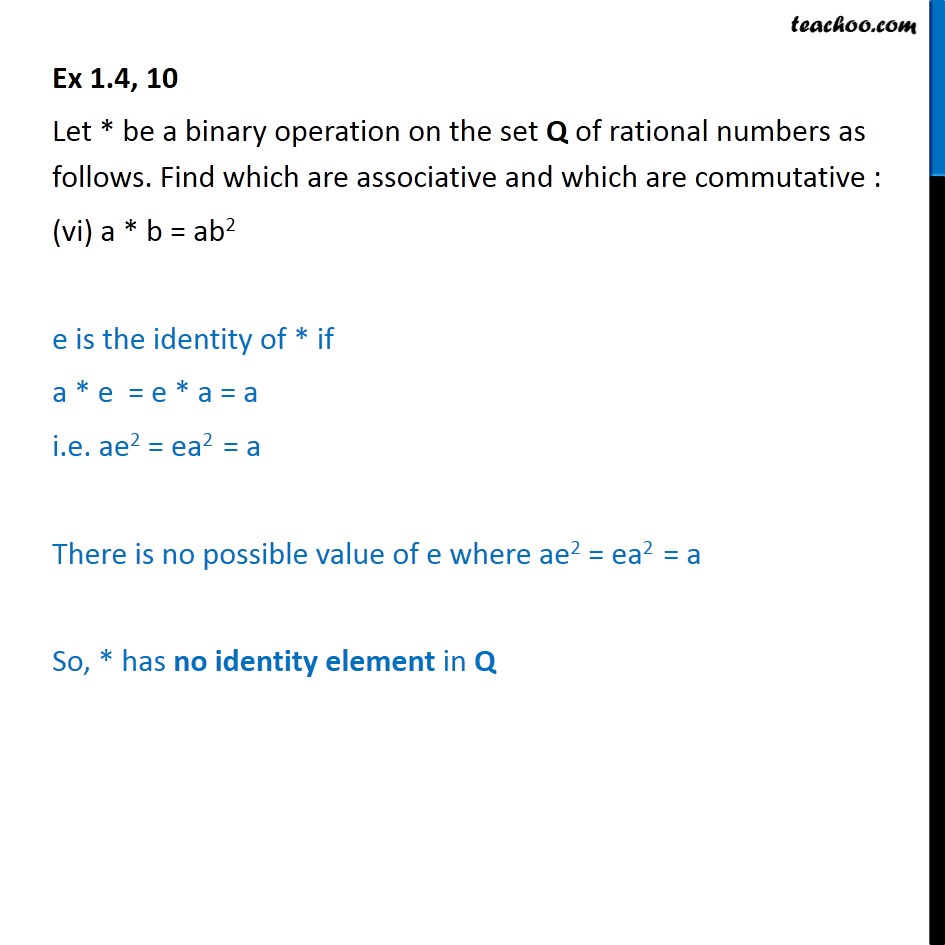Learn in your speed, with individual attention - Teachoo Maths 1-on-1 Class

### Transcript

Ex 1.4, 10 Find which of the operations given above has identity. a * b = a b e is the identity of * if a * e = e * a = a i.e. a e = e a = a There is no possible value of e where a e = e a So, * has no identity element in Q Ex 1.4, 10 Let * be a binary operation on the set Q of rational numbers as follows. Find which are associative and which are commutative : (ii) a * b = a2 + b2 e is the identity of * if a * e = e * a = a i.e. a2 + e2 = e2 + a2 = a There is no possible value of e where a2 + e2 = e2 + a2 = a So, * has no identity element in Q Ex 1.4, 10 Let * be a binary operation on the set Q of rational numbers as follows. Find which are associative and which are commutative : (iii) a * b = a + ab e is the identity of * if a * e = e * a = a i.e. a + ae = e + ae = a There is no possible value of e where a + ae = e + ae = a So, * has no identity element in Q Ex 1.4, 10 Let * be a binary operation on the set Q of rational numbers as follows. Find which are associative and which are commutative : (iv) a * b = (a b)2 e is the identity of * if a * e = e * a = a i.e. (a e)2 = (e a)2 = a There is no possible value of e where (a e)2 = a So, * has no identity element in Q Ex 1.4, 10 Let * be a binary operation on the set Q of rational numbers as follows. Find which are associative and which are commutative : (v) a * b = ab/4 e is the identity of * if a * e = e * a = a i.e. ae/4 = ea/4 = a Which is possible if e = 4 So, 4 is the identity element of * Ex 1.4, 10 Let * be a binary operation on the set Q of rational numbers as follows. Find which are associative and which are commutative : (vi) a * b = ab2 e is the identity of * if a * e = e * a = a i.e. ae2 = ea2 = a There is no possible value of e where ae2 = ea2 = a So, * has no identity element in Q